# Number Line

A number line is a visual representation showing how numbers are arranged on a horizontal straight line.

The arrangement is such that a number to its left is always less than a number to its right. In another way, the numbers on the right are always less than those on the left.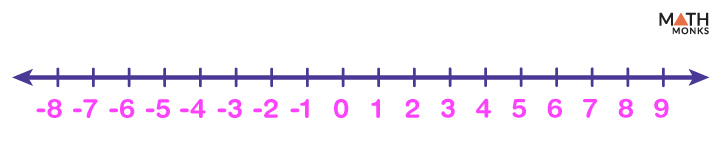Find where are 9 and (-5) on the given number line.

Solution: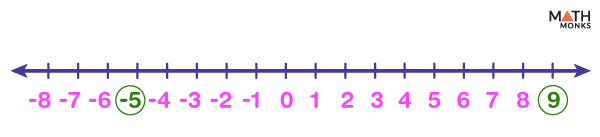The numbers on a number line always increase by one unit to the right or decrease by one unit to the left, as shown.

## Parts

A number line has three parts:

1. 0 – the center point {0}
2. Positive numbers start from 1 and continue forever to the right side {1, 2, 3, 4, 5……}
3. Negative numbers start from -1 and continue forever to the left {………-5, -4, -3, -2, -1}

The numbers on a number line are sequentially placed at equal intervals along its length. It extends infinitely in any direction.

Sometimes number lines have specified starting and closing points known as closed number lines. The interval, including both ends, is called a closed interval.

In contrast, a number line with no numbers marked is called a blank number line.

A number line is usually represented horizontally but can be vertical (y-axis in Cartesian coordinate plane).

## Finding Absolute Value

On a number line, the absolute value is calculated by measuring the distance of that number from zero, irrespective of the direction (left or right). The absolute value always gives a positive number.

For example, 5 is 5 units away from zero. In contrast, (-5) is also 5 units away from zero but in the opposite direction compared to 5.

However, for both 5 and (-5), the absolute value is 5.

## Rational Numbers on a Number Line

Like integers and whole numbers, we can also represent other real numbers like rational (fractions, decimals).

### Fractions on a Number Line

We can represent fractions on a number line by dividing the range between two numbers into some required equal segments. Here, we show the fractions between 0 and 2 when each range (0 to 1 and 1 to 2) is divided into 4 equal parts.

### Decimals on a Number Line

Similarly, we can show decimals on a number line by dividing the range between the two numbers into 10 equal segments.

Here we show the decimal points between 0 and 1 on a number line.

## Irrational Numbers on a Number Line

Like rational numebers, we can also plot irrational numbers on a number line.

For example, let us find the square root of 48 on a number line.

Here we write the whole numbers from 0 to 9 in their square root forms on the number line. As ${\sqrt{48}}$  < ${\sqrt{49}}$ = 7 and it lies between ${\sqrt{36}}$ = 6 and ${\sqrt{49}}$ = 7 so we get 48 on the number line as shown below.

## Using the Number Line

Number line helps us to visualize number sequences and develop strategies for counting and performing arithmetic operations like addition, subtraction, multiplication, and division.

### Comparison

A number line helps us decide if a number is greater than, less, or equal to another number.

For example, consider any random number on a number line, say 8, and choose another number to its right, say 18. Now drawing a number line from 0 to 20, we can see that 18 > 8.

Compare (–15) and 9 using a number line.

Solution: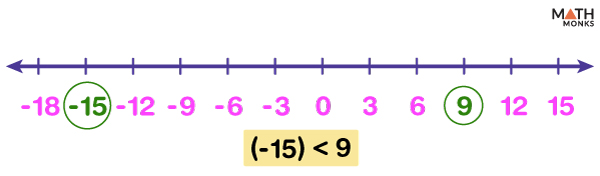We first locate (-15) and 9 on the number line.
Here we draw the number line by plotting the first few multiples of 3.
As we know, any number to the left of 0 is less than any number to the right of 0, so here, (-15) < 9

While adding two numbers, we move to the right side of a number line as many steps as the second number is from the first.

Add 2 and 5 using a number line.

Solution: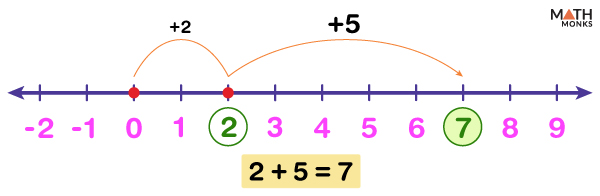Here we locate the first number, i.e., 2, and move 5 steps to the right side from 2 to get the answer. Thus we get the answer that is 7.

### Subtraction

Similar to addition, we move toward the left side of a number line while subtracting two numbers.

Subtract 4 from 10 using a number line.

Solution: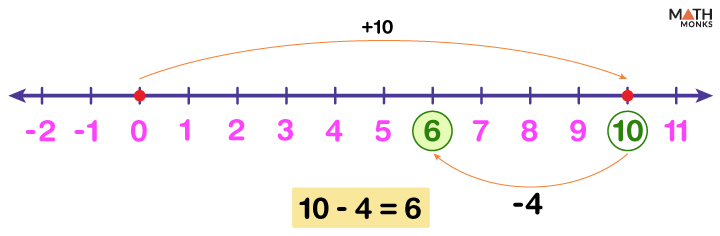Here we locate the first number, i.e., 10, and next, we move 4 steps to the left of 10 to get the result. Thus we get the answer 6

### Multiplication

Since multiplication is also known as repeated addition, we perform addition for the required number of times to get the result.

Find 3 × 4 using a number line.

Solution: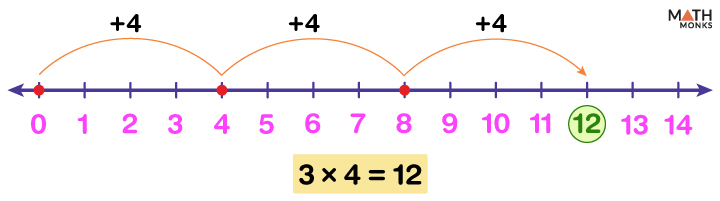Here, 3 × 4 requires the addition of 4 three times to itself, i.e., 3 × 4 = 4 + 4 + 4
First, we locate 4 on the number line. Then, we skip counting on it to show jumps (3 times) of equal size (here 4) following our addition rules. Thus we get the answer 12.

### Division

Since division is also known as repeated subtraction, we perform subtraction to get the result.

We start at the given first number (the dividend) and go backward the number of steps based on the second number (the divisor) each time until we get 0. The number of times we repeat the process gives the quotient of the two numbers.

Find 25 ÷5 using a number line.

Solution: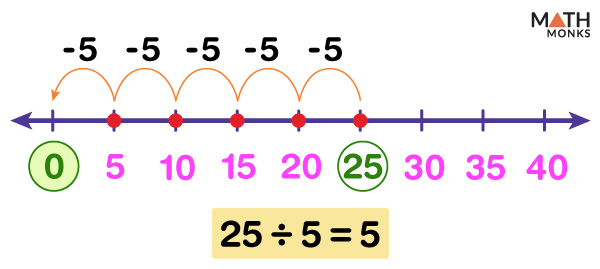We start at the given first number, i.e., 25, then go 5 steps back each time until we get 0. Here, we have plotted the first few multiples of 5 starting from 0, which includes the dividend 25.
Thus, we repeat 5 times the same step to the right of 0 to get 5.

## Skip Counting

Skip counting is a technique to count in intervals other than 1 on a number line. For example, we can skip count by 2 or 3, or 5. It helps us count quickly and represent our results more efficiently on a number line.

Thus, skip counting by x adds x to each previous number to obtain the following number in the series, where x is any whole number.

Show skip counting by 3 starting from 0 on a number line.

Solution: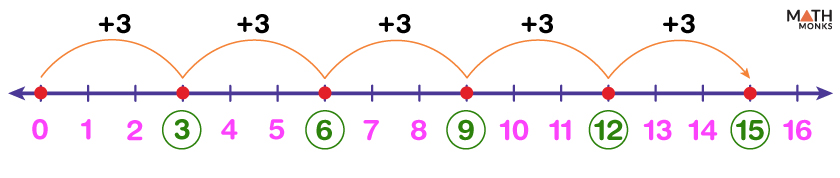Here we start at 0 and skip count by 3. Thus, the series continues like 0, 3, 6, 9, 12, 15 etc.

## Inequalities on a Number Line

Inequalities of form x≤a, x<a, x≥a, or x>a are used to compare two or more numbers. We can graph the inequality on a number line and then identify the shaded values that satisfy the inequality.